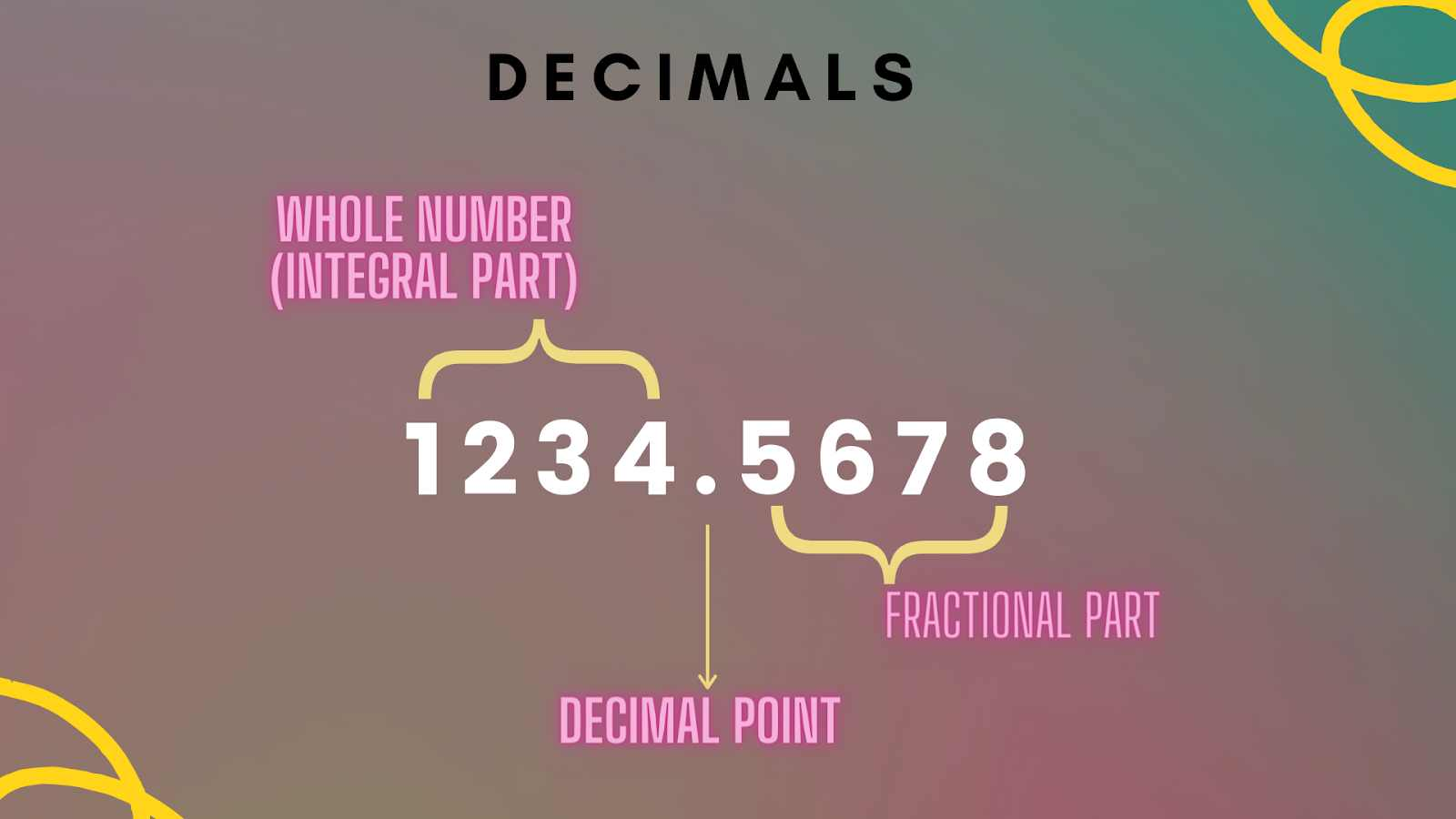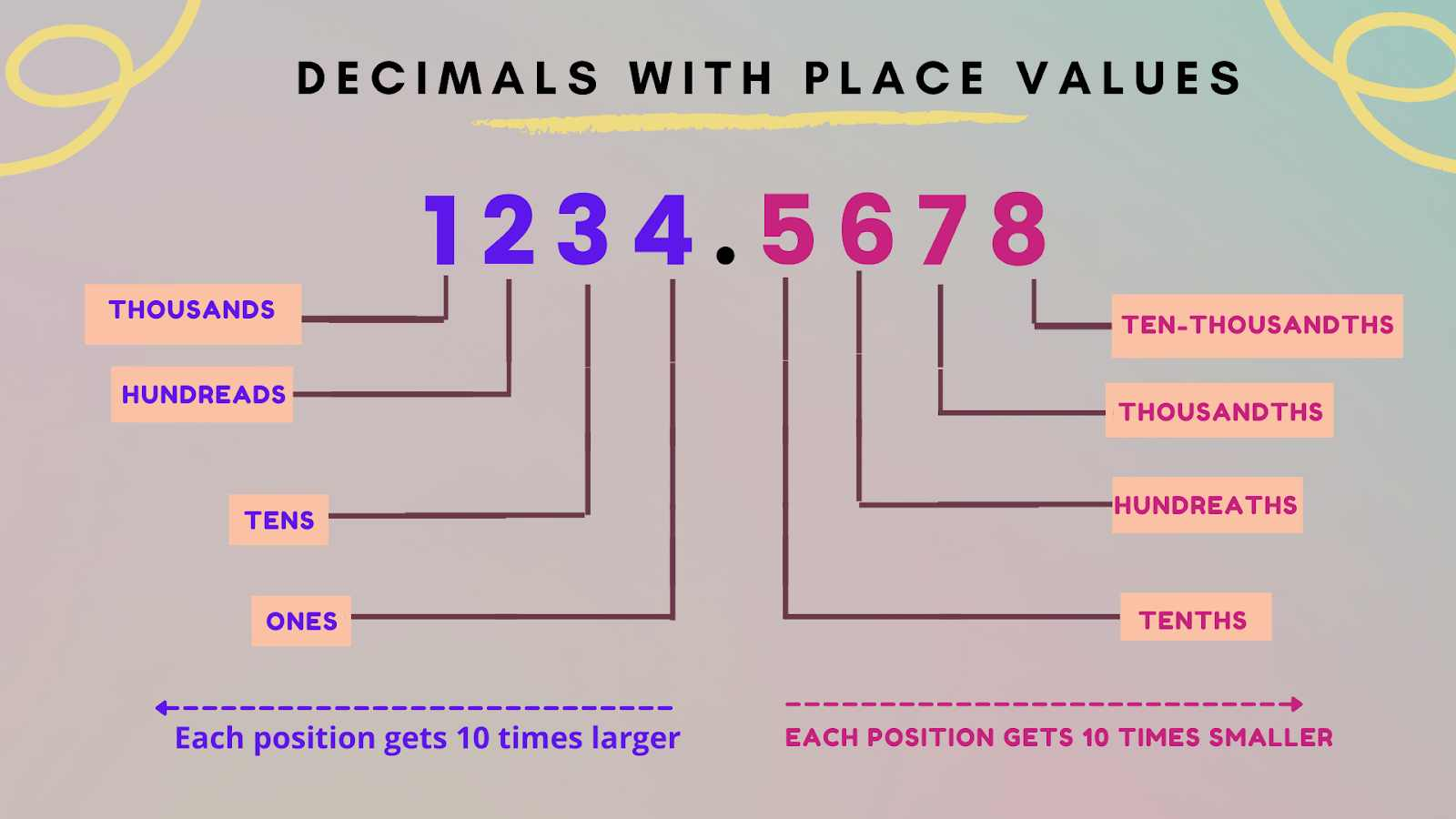Courses
Courses for Kids
Free study material
Offline Centres
More

# DecimalsLast updated date: 26th Nov 2023
Total views: 140.1k
Views today: 3.40k## Understanding Decimal Number System

On a number line, decimals are a set of numbers that lie between integers. They are simply another way to represent fractions. We can write more precise values of measurable quantities such as length, weight, distance, money, etc using decimals.

The numbers to the left of the decimal point are integers or whole numbers, while the numbers to the right are decimal fractions. In this article, we will learn about decimals.

## Decimals Number System

The decimal number system is a positional notation that has a base of 10. It refers to numbers with a fractional part separated from an integer part by a decimal separator. Decimals allow us to write fractions without having to write a fraction with a numerator and denominator.The above image Illustrates the Parts of a Decimal Number

## Decimal Place Value Chart

The position of each digit is important when writing any number. When we go to the right in the below place value chart, the value of the digit becomes 10 times smaller, i.e., one-tenth, and when we move to the left, the value of the digit becomes ten times larger.The above Decimals images Illustrate a Decimal Number with Corresponding Place Values

## Types of Decimals

Decimals are categorised based on the type of digits that follow the decimal point. It depends on whether the digits are non-terminating or terminating.

Examples:

• Non-terminating: 122.353535.... , 3.33333...., 13.66666…

• Terminating: 85.9856, 2.5674, 7.5

## Conclusion

The decimal numeral system is a standard system for showing integer and non-integer numbers. You can't learn about numbers completely unless you understand decimals. Hopefully, this article has offered you a good understanding of the significance of decimals.

## FAQs on Decimals

1. Why are decimals important?

We use decimals all the time while dealing with money, weight, length, and so on. Decimal numbers are used when greater precision is required than whole numbers provide. For example, if we calculate our weight on the weighing machine, we may not always find the weight equivalent to a whole number on the weighing scale. In such conditions, the decimal system helps us to get precise values.

2. Why is the decimal point so important?

The decimal point divides the whole number from the fractional part of the number. In 128.87, the decimal point will separate the whole number 128 from fractional part 87. The decimal point makes a decimal number easy to read.

3. Where do we use decimals in everyday life?

When dealing with money, the usage of decimals is important. Whenever there is a purchase or sale, decimals are used. The price of products is not always expressed in whole numbers. In such situations, you'll need to know how to understand and add the given values. So, decimal knowledge is required. Decimals are also used to measure the length, weight, etc.